Mobile QR Code1. (School of Electrical and Electronics Engineering, Chung-Ang University, Seoul 06974, Korea)
2. (Electronics and Telecommunications Research Institute (ETRI), Daejeon 34129, Korea)

Analog-to-digital converter, successive approximation register, oversampling, noise shaping, mismatch error shaping

## I. INTRODUCTION

Modern IoT devices demand high resolution, power efficient ADCs for sensor interfaces such as human body communication, edge computing, and biomedical sensors. Successive approximation register (SAR) ADCs are architectures that are well known for their technology scalability. They are also recognized for their power and area efficiency due to their digitally based building blocks. However, realizing high resolution while maintaining the advantages of SAR ADCs is problematic due to thermal noise such as kT/C and comparator noise. This is because in order to mitigate such noise, the resolution of the CDAC must be increased. Additionally, a low-noise comparator that consumes a lot of power is also required. In the case of CDAC, total capacitance increases exponentially with resolution, which occupies a large area while increasing the power consumption of the driving buffer as well. Given these factors, the benefits of SAR ADCs tend to diminish with increasing resolution.

On the other hand, delta-sigma modulation (DSM) architectures are traditionally regarded as the most promising candidates for high-resolution ADCs due to their oversampling and noise shaping (NS) characteristics. While these noise reduction effects do indeed improve SNR performance by suppressing overall noises, the disadvantage is that DSM architectures require power-hungry op amps, which occupy large areas and cannot easily scale with technology. Furthermore, flash ADC used as a multi-bit quantizer in DSM architectures typically offers lower resolutions, which in turn require a higher oversampling ratio (OSR) to achieve high SNR. It leads to a decrease in power efficiency.

Therefore, NS-SAR ADC, a hybrid architecture that combines DSM and SAR ADC to achieve high SNR while maintaining both advantages, has become a promising candidate for high-resolution ADCs in recent years [1-41]. In this hybrid architecture, the SAR ADC is used as a multi-bit quantizer and concurrently as a feedback DAC which processes the residue to achieve noise shaping performance.

Fig. 1 compares the NS-SAR ADCs with conventional SAR and DSM ADCs, which have been published in major conferences . The solid line and dashed line indicate 180 dB of Schreier Figure-of-Merit (FoM$_{\mathrm{S}}$ as shown in Eq. (1)) and 5 fJ/c.-s of Walden FoM (FoM$_{\mathrm{W}}$ as shown in Eq. (2)), respectively. It shows that NS-SAR ADCs are pioneers in terms of power efficiency, as compared with conventional architectures. Reference [36,37] have reviewed the NS-SAR ADC to show the development of this architecture.

##### (1)
$FoM_{S}=SNDR+10\log \left(\frac{f_{B}}{power}\right)$
##### (2)
$FoM_{W}=\frac{power}{2^{\left(SNDR-1.76\right)/6.02}\times 2\times f_{B}}$

In this paper, the fundamentals of the NS and the advances in noise reduction techniques for NS-SAR ADCs are reviewed. In particular, the loop filter implementations have been described in depth. The rest of this paper is organized as follows. Section II provides the basic concept of NS-SAR ADCs including oversampling and NS mechanisms. Section III outlines the noise reduction techniques employed to realize NS characteristics with residue processing loop filters while Section IV introduces kT/C noise cancellation and CDAC mismatch error shaping (MES). Finally, Section V presents the advanced architectures employed to overcome the limitations of NS-SAR ADCs and Section VI concludes this paper.

##### Fig. 1. Power efficiency comparison between NS-SAR ADCs with conventional architectures.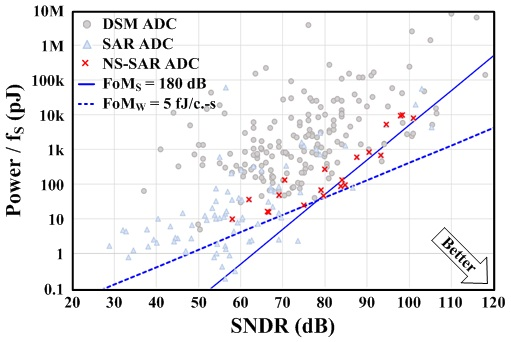## II. BASIC OF NOISE-SHAPING (NS) SAR ADCS

### 1. Oversampling

Oversampling is a common method of improving the SNR by reducing quantization noise. The oversampling ADC samples and quantizes the input signal at a much higher sampling rate than the signal band. Therefore, a small fraction of quantization noise falls into the signal band making it possible to filter the out-band noise as shown in Fig. 2. The reduction of quantization noise by oversampling can be quantified as Eq. (3). For better understanding, the signal-to-quantization noise ratio (SQNR) is considered rather than SNR.

##### (3)
$SQNR=10\log \left(\frac{P_{signal}}{P_{q,noise}}\right)+10\log \left(OSR\right)$

where $P_{signal}$ and $P_{q,noise}$ denote signal and quantization noise power, respectively. The OSR is the ratio between the signal band ($f_{B}$) and Nyquist range ($f_{S}/2$). According to the above equation, there is only a 3-dB improvement in SQNR when OSR is doubled. Therefore, a very high OSR is required for high-resolution ADC designs, which has the effect of degrading power efficiency. To overcome this limitation, NS schemes are widely used along with oversampling.

##### Fig. 2. Quantization noise and noise transfer function (NTF).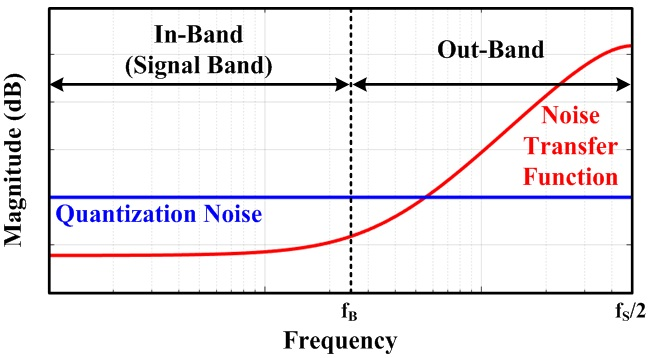### 2. Noise Shaping (NS)

Noise shaping is an essential technique and a basic concept of oversampling ADCs to enhance noise reduction effects. This is achieved by attenuating in-band noise through a high-pass noise transfer function (NTF) as shown in Fig. 2. The 1st-order DSM can be an example for presenting the NS mechanism.

The 1st-order DSM consists of a quantizer, a feedback DAC, and an integrator as described in Fig. 3. The quantizer digitizes analog input with feedback DAC converting this digitized data to an analog signal and subtracting from input, making residue voltage that contains quantization noise. Eq. (4) shows a transfer function including quantization noise, $Q\left(z\right)$.

##### (4)
$D_{OUT}\left(z\right)=\frac{H\left(z\right)}{1+H\left(z\right)}V_{IN}\left(z\right)+\frac{1}{1+H\left(z\right)}Q\left(z\right)$

If the $H\left(z\right)$ is a simple discrete-time integrator, $H\left(z\right)=z^{-1}/\left(1-z^{-1}\right),$ then $D_{OUT}\left(z\right)$ can be expressed as follows.

##### (5)
$D_{OUT}\left(z\right)=z^{-1}V_{IN}\left(z\right)+\left(1-z^{-1}\right)Q\left(z\right)$

Eq. (5) shows a simple delay signal transfer function (STF), $z^{-1}$, and a high-pass NTF, $1-z^{-1}.$ Therefore, the SQNR including in-band noise attenuation by NTF can be expressed as Eq. (6).

##### (6)
$SQNR=10\log \left(\frac{P_{signal}}{P_{q,noise}\times IBNG}\right)+10\log \left(OSR\right)$

Note that IBNG denotes in-band noise gain, which is $IBNG=~ \int _{0}^{f_{B}}\left(\left| NTF\left(f\right)\right| ^{2}/f_{B}\right)df$. If NTF is assumed to be $1-z^{-1}$, Eq. (6) can be approximated as follows.

##### (7)
$SQNR\approx 10\log \left(\frac{P_{signal}}{P_{q,noise}}\right)-10\log \left(\frac{\pi ^{2}}{3}\right)+30\log \left(OSR\right)$

Eq. (7) shows that the SQNR increases 9-dB when OSR is doubled. Fig. 4 compares the SQNR improvement between oversampling with and without NS.

##### Fig. 3. (a) Block diagram of the 1st-order DSM; (b) signal flow diagram.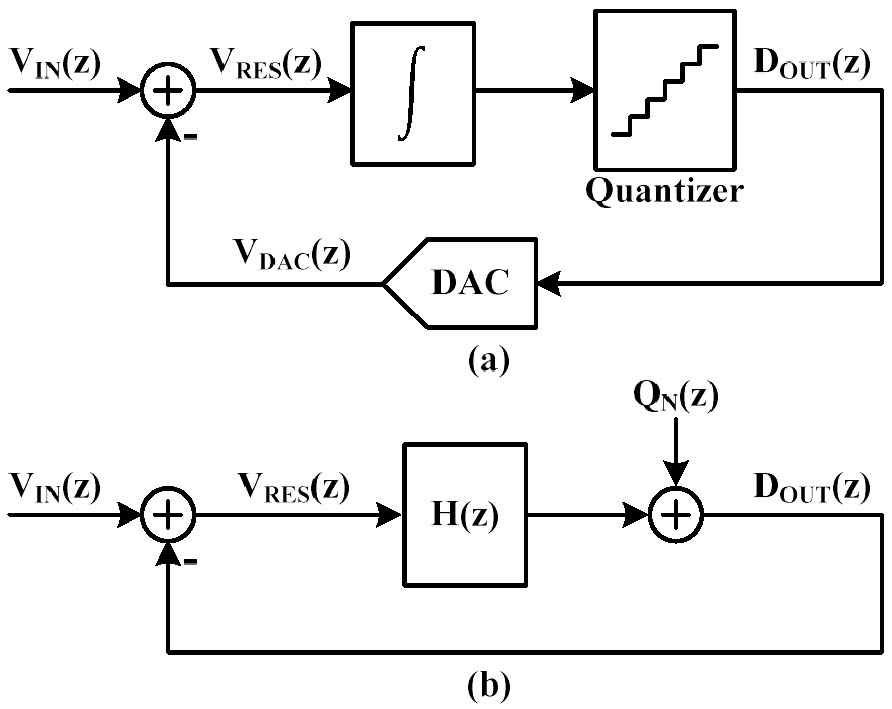##### Fig. 4. SQNR improvement by oversampling with and without NS.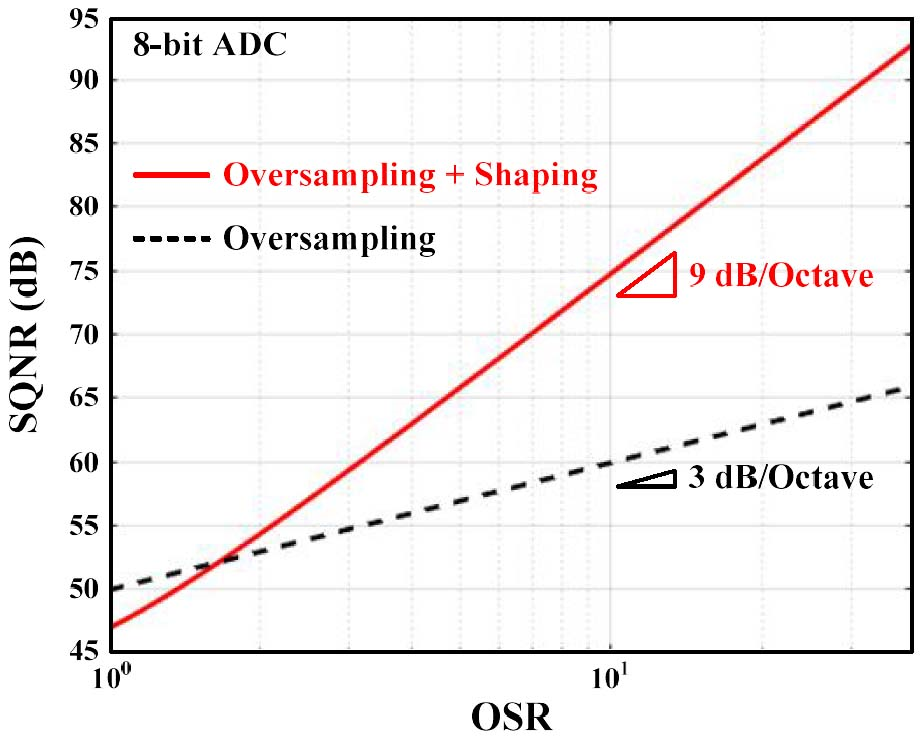### 3. Noise-shaping (NS) SAR ADCs

NS-SAR ADCs have been proposed as a means to overcome the shortcomings of DSM ADCs while obtaining similar NS characteristics . The proposed architecture replaces a quantizer and a feedback DAC of CIFF-DSM as a SAR ADC as shown in Fig. 5(a). And it realizes the NS using a finite-impulse-response and infinite-impulse-response (FIR-IIR) loop filter. The switched-capacitor FIR filter samples the residue and IIR filter integrates this residue. The signal-flow diagram including the FIR-IIR loop filter can be modeled as in Fig. 5(b). Therefore, the overall transfer function is given by Eq. (7).

##### (7)
$D_{OUT}\left(z\right)=V_{IN}\left(z\right)+\frac{1}{1+A\left(z\right)B\left(z\right)}Q\left(z\right)$

Even though  achieves a sharp NTF as shown in Fig. 6, the SNDR is limited due to additional noise generated by the FIR-IIR filter. Furthermore, a power-consuming amplifier is still required. To overcome these limitations, various noise reduction techniques have been proposed. In this paper, the NS-SAR ADCs are classified into active and passive topologies based on the loop filter implementations, which are reviewed in more detail in the following section.

##### Fig. 5. (a) Block diagram of NS-SAR ADC; (b) signal flow diagram.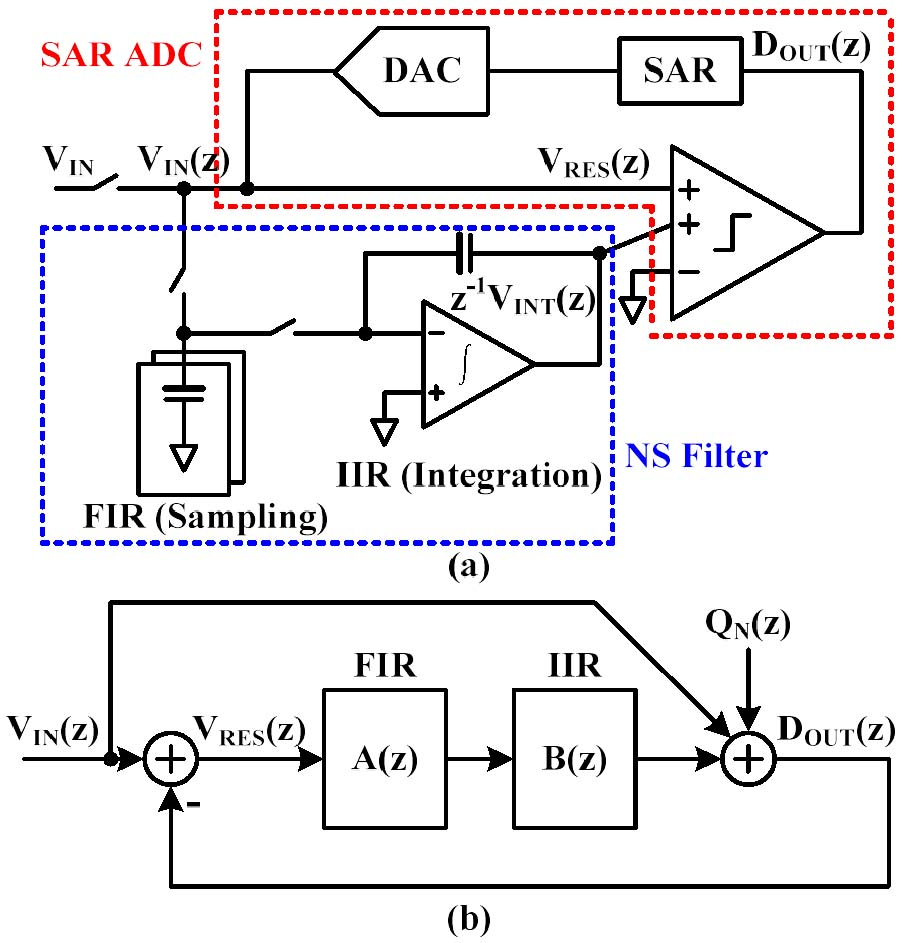##### Fig. 6. NTF comparison between NS-SARand 1st-order DSM ADC.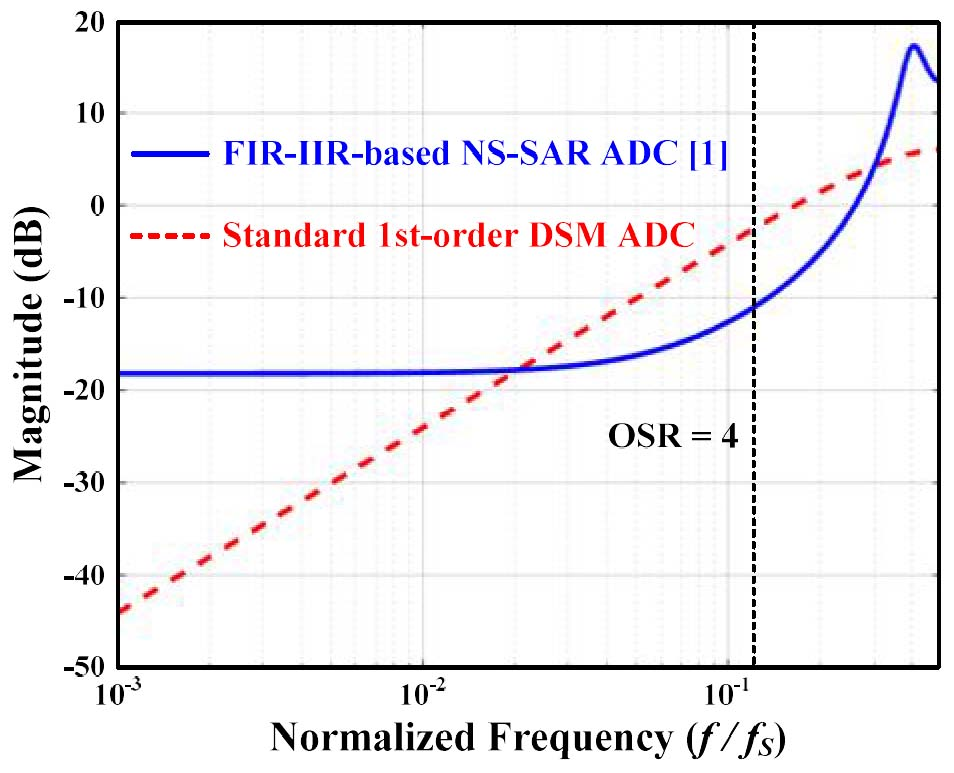## III. NOISE REDUCTION TECHNIQUES WITH RESIDUE PROCESSING

The most important factor of NS-SAR ADCs is how to extract the residue and sum it with the following input signal. There are thus two concerns 1) how to process the residue and 2) how to implement the loop filter. With regard to residue processing, both cascaded-integrator-feed-forward (CIFF) and error-feedback (EF) are common implementations. In general, the CIFF structures have a feed-forward path to process the residue and sum it to the following input using a multi-input comparator, whereas the EF structures have a feedback path and sum the residue to the input voltage directly on CDAC. Note that the multi-input comparator introduces extra noise in CIFF and the charge-sharing summation in EF induces signal attenuation.

Fig. 7(a, b) and Fig. 8(a, b)shows signal flow diagrams and circuit implementations of CIFF and EF structures, respectively. The corresponding transfer functions are as follows.

##### (8)
$D_{OUT}\left(z\right)=V_{IN}\left(z\right)+\frac{1}{1+H\left(z\right)z^{-1}}Q_{N}\left(z\right)$
##### (9)
$D_{OUT}\left(z\right)=V_{IN}\left(z\right)+\left(1-E\left(z\right)z^{-1}\right)Q_{N}\left(z\right)$

From the circuit implementation of each structure, Eq. (8) and (9) can be translated to Eq. (10) and (11), respectively.

##### (10)
$D_{OUT}\left(z\right)=V_{IN}\left(z\right)+\frac{1-0.64z^{-1}}{1+1.28z^{-1}+0.64z^{-2}}Q\left(z\right)$
##### (11)
$D_{OUT}\left(z\right)=\frac{14}{16}V_{IN}\left(z\right)+\left(1-\frac{30}{16}z^{-1}+\frac{30}{32}z^{-2}\right)Q\left(z\right)$

Note that, the CIFF structure needs a high-gain integrator to achieve ideal high-pass NTF. On the other hand, the EF structure does not require a high gain integrator but requires a high accuracy opamp. This is because the gain of opamp directly controls the zero of NTF.

Thus, while there are many efforts to alleviate the burden of high gain integrator and multi-input comparator in CIFF structure such as passive integration and capacitor stacking, gain calibrations are introduced to precisely control the gain of opamp in EF structures. Table 1. summarizes and compares the CIFF and EF structures.

In terms of loop filter implementation, the loop filter for realizing the NTF of each structure is implemented in two ways, namely active and passive topologies. Generally, an active strategy uses op amps whereas the passive strategy uses simple switches and capacitors to process the residue. More details of these loop filters are provided in the following sections.

##### Fig. 7. (a) Signal flow diagram; (b) circuit implementationof CIFF structures.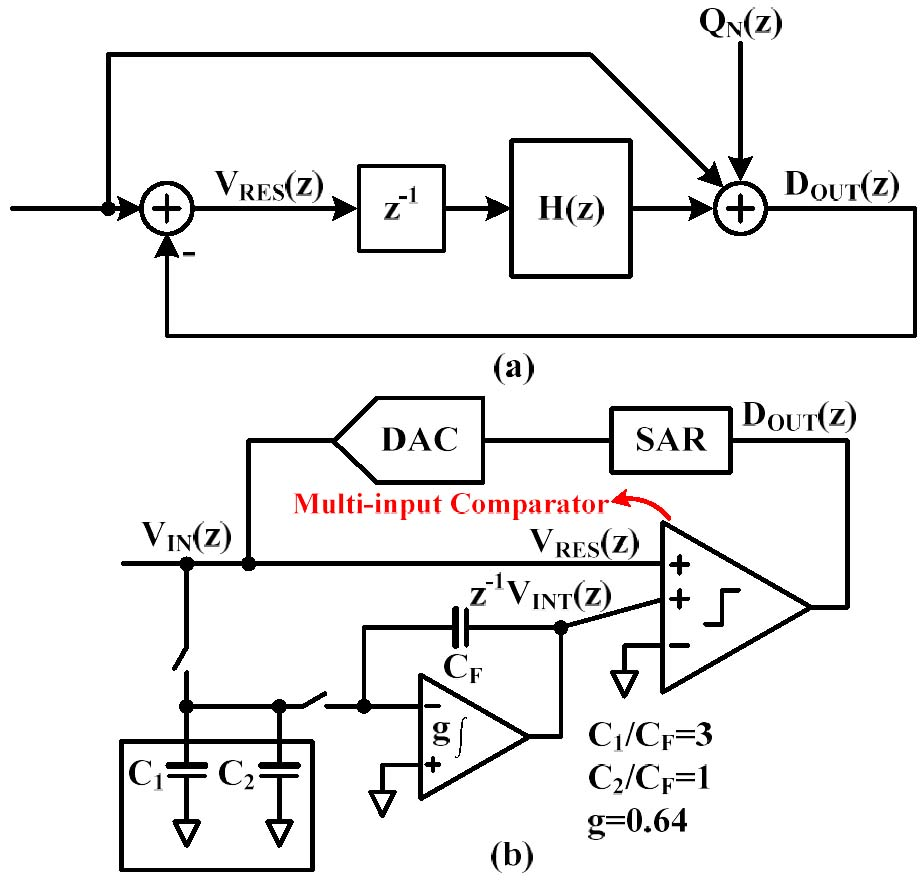##### Fig. 8. (a) Signal flow diagram; (b) circuit implementationof EF structures.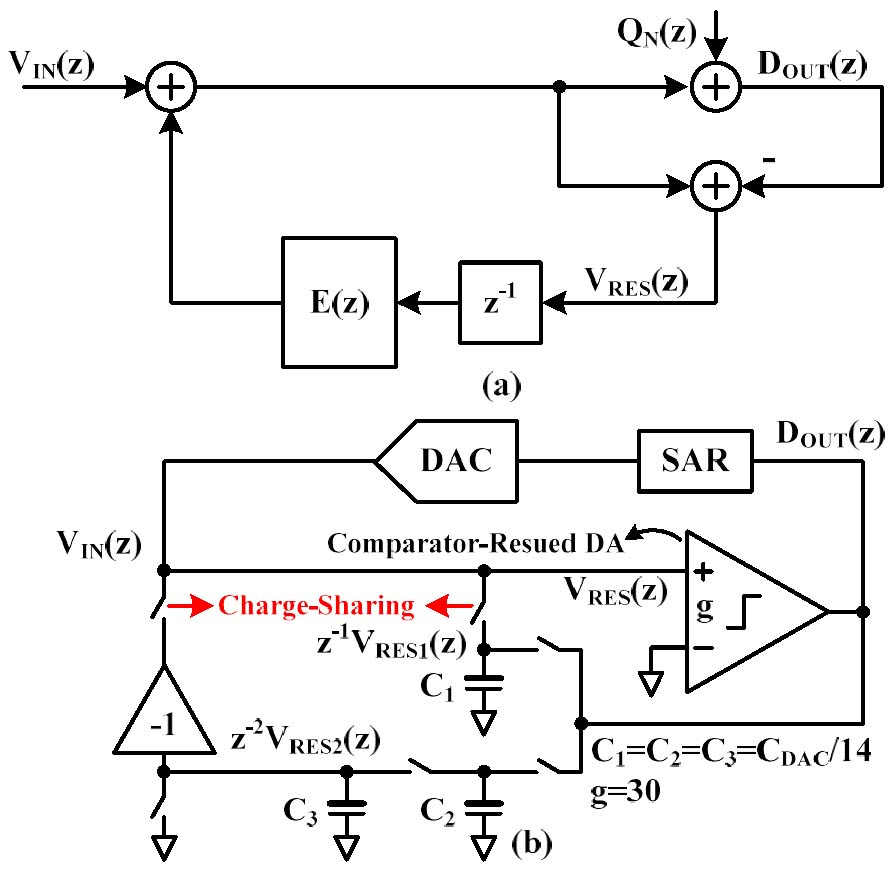##### Table 1. Comparison between CIFF and EF
 Structure Residue Summation Gain Gain Sensitivity Limitation CIFF Multi-input comparator High Low Extra noise EF Charge-sharing Medium High Signal attenuation

### 1. Active Loop Filter

Active loop filters show flexible and sharp NTF because the amplifier provides sufficient gain. However, the active amplifier consumes large amounts of power which degrades the efficiency of NS-SAR. Therefore, much effort has been expended in a bid to reduce the power consumption of active strategies.

As discussed in the previous section, the first implementation of NS-SAR ADC is an active strategy using the FIR-IIR loop filter proposed in . The switched-capacitor FIR filter and the active-amplifier-based IIR filter samples and integrates the residue, respectively. However, the proposed architecture presents only moderate SNDR performance, even though it has sharp NTF, because the passive residue sampling introduces considerable kT/C noise, with the active amplifier also introducing more noise. Improving noise performance requires the addition of large capacitors for residue sampling, while high-gain, power-consuming amplifiers are required in the IIR filter if the additional noise is to be mitigated.

To relieve the trade-off between kT/C noise and gain loss due to charge sharing in switched-capacitor FIR filter, an input buffer, implemented as an open-loop amplifier between CDAC and residue sampling capacitor, is required  as shown in Fig. 9. Although it provides a gain of 2 alleviating kT/C noise in the FIR filter, the input buffer and active amplifier in the IIR filter together consume 37% of the total power.

In [10,11], the open-loop dynamic-amplifier-based FIR-IIR filter is proposed as a means of reducing these extra power consumptions. Reference  replaces the input buffer and active integrator with low power open-loop dynamic amplifiers and  proposes a gain-enhanced dynamic amplifier as the input buffer. Moreover,  greatly reduces the residue sampling capacitor, such that the active-amplifier-based IIR filter can be substituted by a simple switched-capacitor integrator as shown in Fig. 10. However, the drawback is that the open-loop dynamic amplifier is sensitive to PVT variation, which degrades the NTF and limits the NS performance. To compensate for this drawback,  proposes background calibration for dynamic amplifiers which increases design complexity.

Reference  proposes a calibration-free PVT-robust closed-loop 2-stage dynamic amplifier to simplify the design complexity of the calibration as illustrated in Fig. 11. Due to the fact that the gain of the closed-loop amplifier is set by capacitor ratios, it is robust in the presence of PVT variations. In  and , the PVT-insensitive voltage-time-voltage converter and PVT-robust source follower-based unit gain buffer for active residue processing are proposed , respectively.

##### Fig. 9. FIR-IIR loop filter with input buffer.##### Fig. 10. FIR-IIR loop filter with dynamic amplifier and switched-capacitor implementation.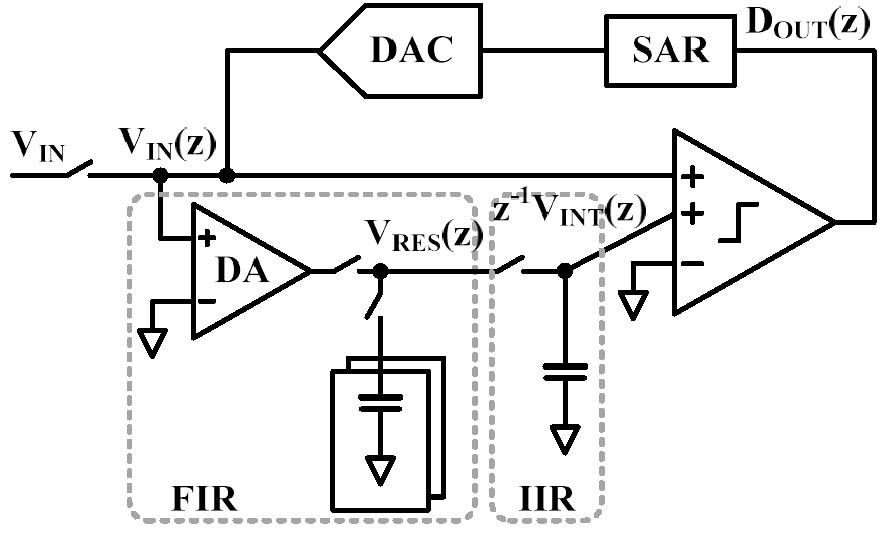##### Fig. 11. PVT-robust closed-loop 2-stage dynamic amplifier.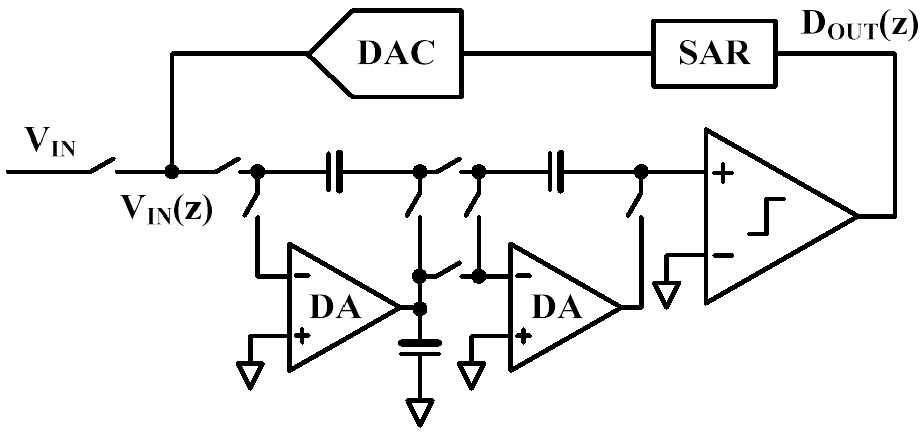### 2. Passive Loop Filter

A passive loop filter is a simple, PVT robust, and scaling-friendly strategy because it consists of switches and capacitors. It is more power-efficient than an active loop filter given that it does not need a power-consuming amplifier. However, the passive strategy suffers from charge sharing in switched-capacitor operation and shows mild NTF due to the insufficient gain. Therefore, many efforts have been expended on reducing the charge sharing and providing adequate gain for the passive loop filter.

The first fully passive NS-SAR ADC is proposed in . The proposed architecture utilizes the switched-capacitor circuit for the purpose of residue sampling and integration. Residue summation is realized by a multi-input comparator as shown in Fig. 12. However, switched-capacitor residue sampling attenuates the input signal by factor of 2 while the charge sharing of residue integration degrades NTF. This architecture is improved in  to 2nd-order NS. The 2nd-order NS is realized with the application of capacitor stacking  thus achieving passive gain but this does nothing to resolve signal attenuation limitations. Reference [4,8] eliminates this signal attenuation and realizes sharper NTF than [2,3]. In addition, the relative gain between input signal and integrated residue is realized through the input transistor sizing of the multi-input comparator as shown in Fig. 13. The multi-input comparator not only provides relative gain but also realizes signal summation. However, the large extra input pair which provides relative gain also introduces additional thermal noise, while the residue sampling and integration introduce extra unshaped kT/C noise, increasing the overall noise.

To alleviate the extra thermal noise from the multi-input comparator,  proposes a passive signal-residue summation scheme as shown in Fig. 14. This architecture achieves the residue summation by serialization of CDAC and integration capacitor. Moreover, the differential residue sampling on back-to-back capacitors provides a passive gain of 2 thereby eliminating the multi-input comparator. Even so, it achieves only 2x passive gain so resulting in mild NTF while the small residue sampling capacitor introduces large kT/C noise.

In , the differential integration with split capacitor and capacitor stacking are presented as described in Fig. 15. This scheme eliminates residue sampling and provides 4x the passive gain, which obviates the need for a multi-input comparator. Therefore, it reduces the kT/C and comparator noise significantly. However, it remains difficult to increase gain because the passive gain using capacitor stacking is sensitive to parasitic capacitance.

##### Fig. 12. Fully passive NS-SAR ADC.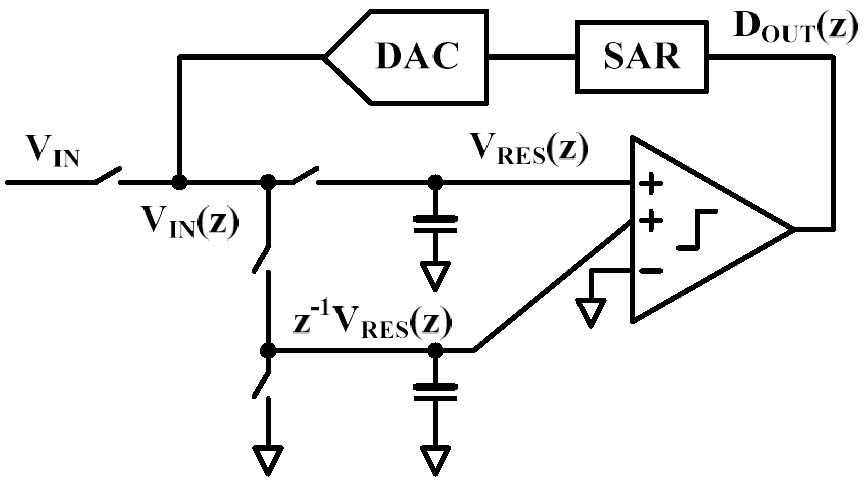##### Fig. 13. Passive NS-SAR ADC with relative gain using multi-input comparator.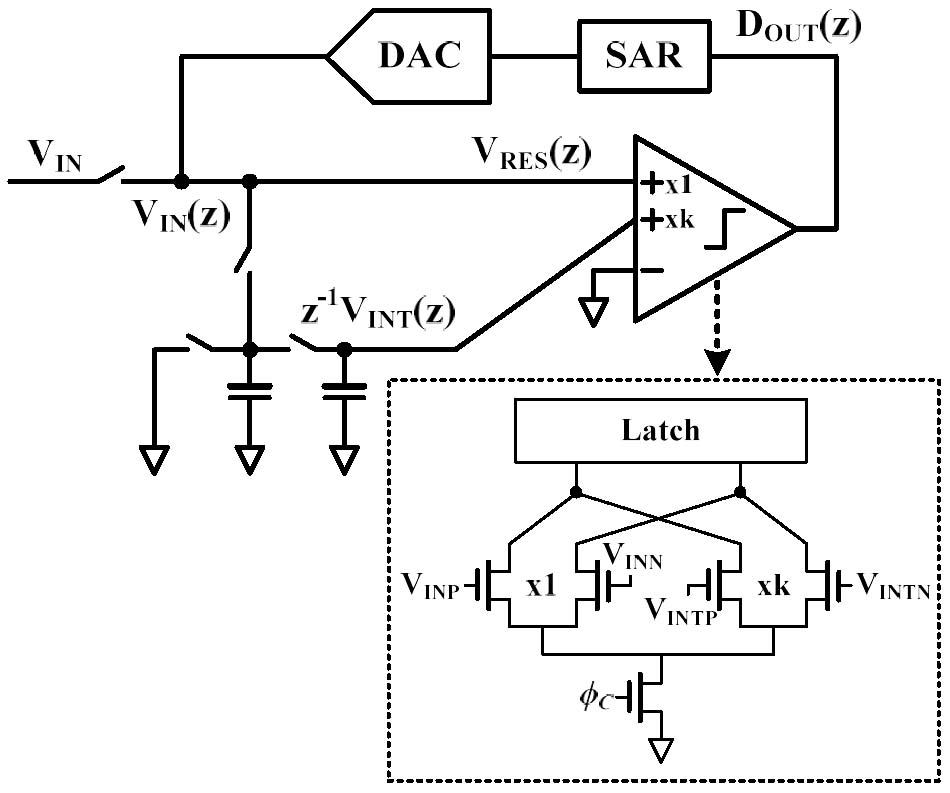##### Fig. 14. Passive signal-residue summation with 2x passive gain using capacitor stacking.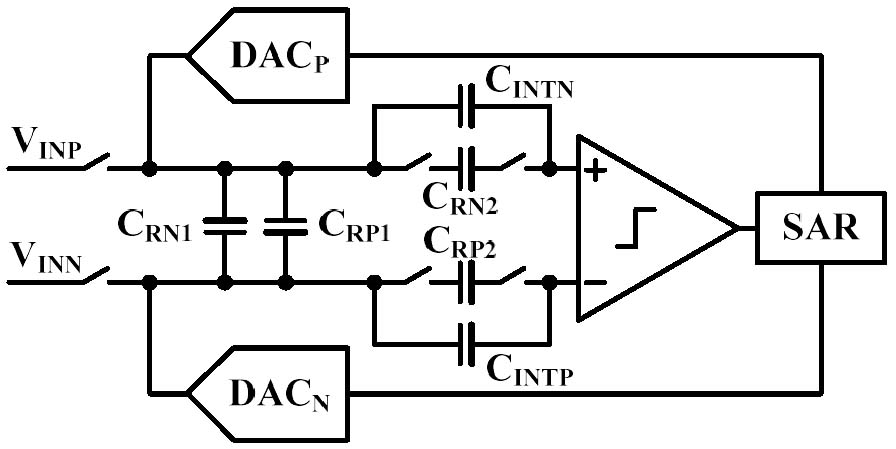##### Fig. 15. Passive residue integration with 4x passive gain using capacitor split and stacking.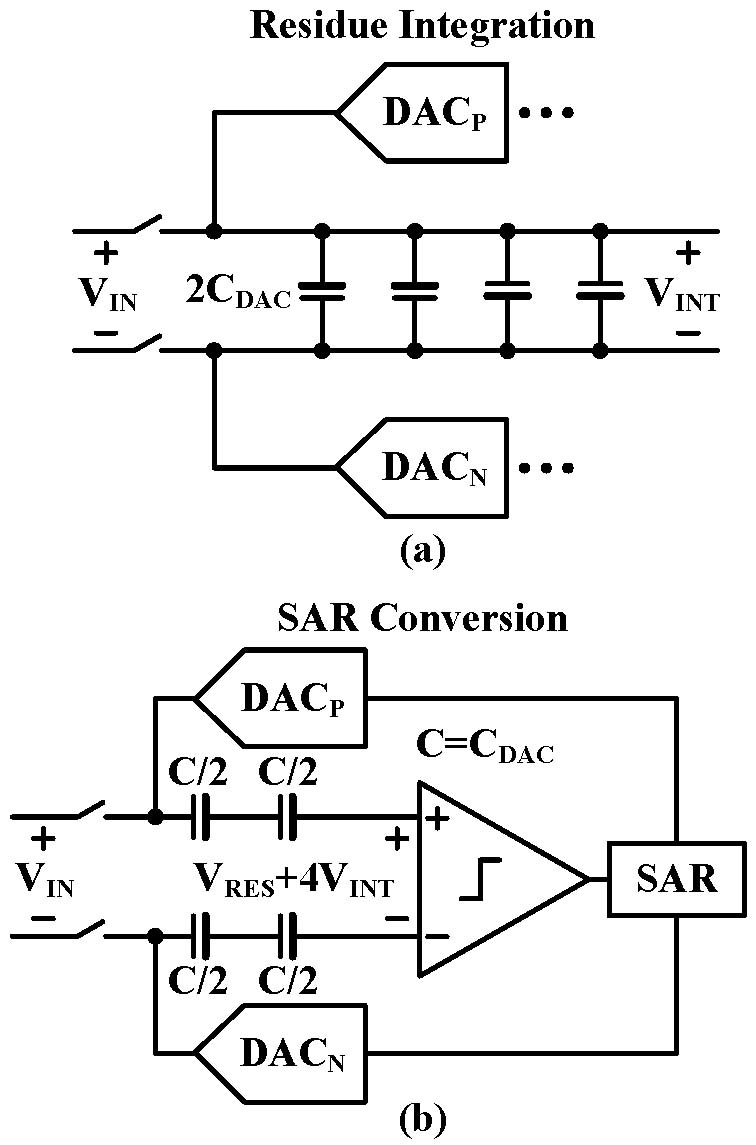## IV. ADDITIONAL NOISE AND ERROR REDUCTION TECHNIQUES

Although the NTF efficiently suppresses quantization and comparator noise, additional noise and error that are not shaped by NTF remain. These additional non-idealities, such as the CDAC mismatch-induced error and kT/C noise, serve to limit the SNDR of the NS-SAR ADCs. This section, therefore, presents the noise and error reduction techniques to mitigate these additional non-idealities.

### 1. CDAC Mismatch

The CDAC mismatch introduces additional unshaped errors which cause harmonic distortion and increase the in-band noise floor. It can be modeled as an additive noise, ε(z), in a loop filter as shown in Fig. 16. Then $D_{OUT}\left(z\right)$ can be expressed as follows.

##### (10)
$D_{OUT}\left(z\right)=V_{IN}\left(z\right)+\frac{1}{1+H\left(z\right)z^{-1}}Q_{N}\left(z\right)+\varepsilon \left(z\right)$
##### (11)
$D_{OUT}\left(z\right)=V_{IN}\left(z\right)+\left(1-E\left(z\right)z^{-1}\right)Q_{N}\left(z\right)+\varepsilon \left(z\right)$

Calibration and mismatch shaping (MS) are commonly used methods to relieve the CDAC mismatch. The first one, calibration, including both the foreground and background method, compensates for CDAC mismatch in the analog and digital domain, and thus it can cancel errors from the CDAC mismatch. Foreground calibration is more widely used than the background method due to the greater simplicity of its implementations. However, foreground calibration needs additional calibration phase interrupting normal operation of ADCs.

The second one, MS suppresses the in-band noise and distortion from CDAC mismatch. The well-known MS are the dynamic elements matching (DEM)  and data-weighted averaging (DWA) . An element selection logic (ESL) randomizes and rotates the DAC capacitor as shown in Fig. 17. Therefore, the DEM and DWA suppresses and shapes the in-band harmonic distortion and noise, respectively. The drawbacks of these DEM-based MS methods are complexity and long delay. If higher order shaping is to be achieved, more complicated logic is required, with ESL complexity growing exponentially as CDAC resolution increases. To limit complexity of the ESL, some designs shuffle only a few MSBs, despite the continuing presence of errors from LSBs.

Another MS technique is mismatch error shaping (MES) . Conceptually, it is similar to the NS mechanism. It captures the mismatch error and feeds it back by presetting the LSBs of CDAC before sampling. Fig. 18 illustrates the 1st-order MES operations. During the sampling phase (in Fig. 18(a)), the LSB parts are preset to hold the previous mismatch error, $z^{-1}E_{M}\left(z\right)$.

Following this, the LSB parts are reset in the conversion phase (in Fig. 18(b)) to subtract the previous error from the current error, $E_{M}\left(z\right)-z^{-1}E_{M}\left(z\right)$. Therefore, it realizes a high pass shaping of the CDAC mismatch error, $\left(1-z^{-1}\right)E_{M}\left(z\right)$. The MES is easier to implement than DEM because it does not require complex logic. However, the MES suffers input dynamic range loss due to LSB presetting.

##### Fig. 16. Signal flow diagram of (a) CIFF; (b) EF structures including noise from CDAC mismatch.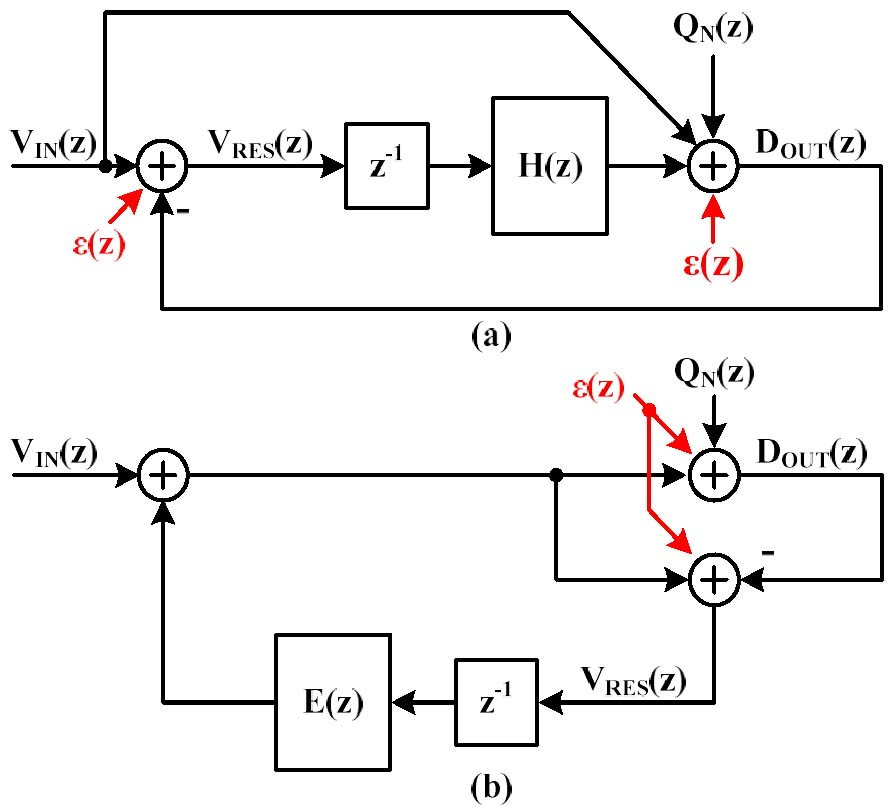##### Fig. 17. Block diagram of a simple DEM.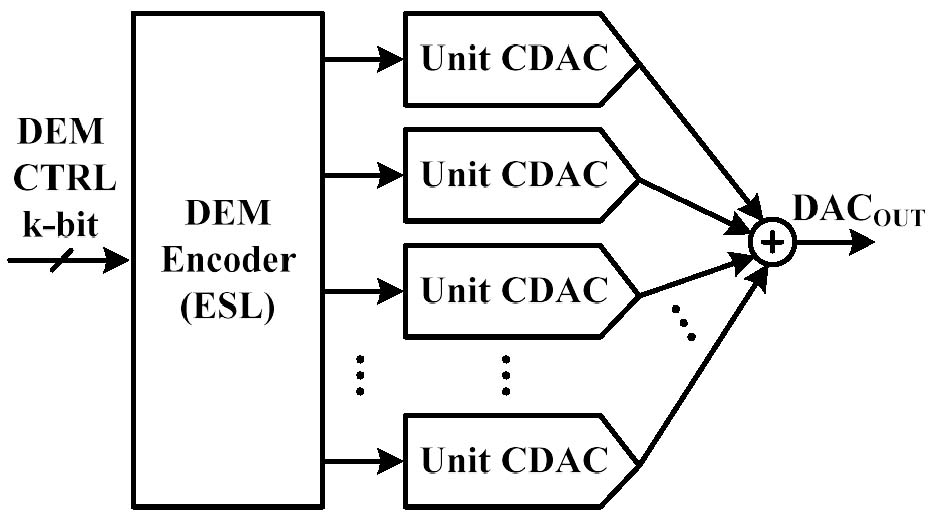##### Fig. 18. Block diagram of a simple MES: (a) sampling phase; (b) conversion phase.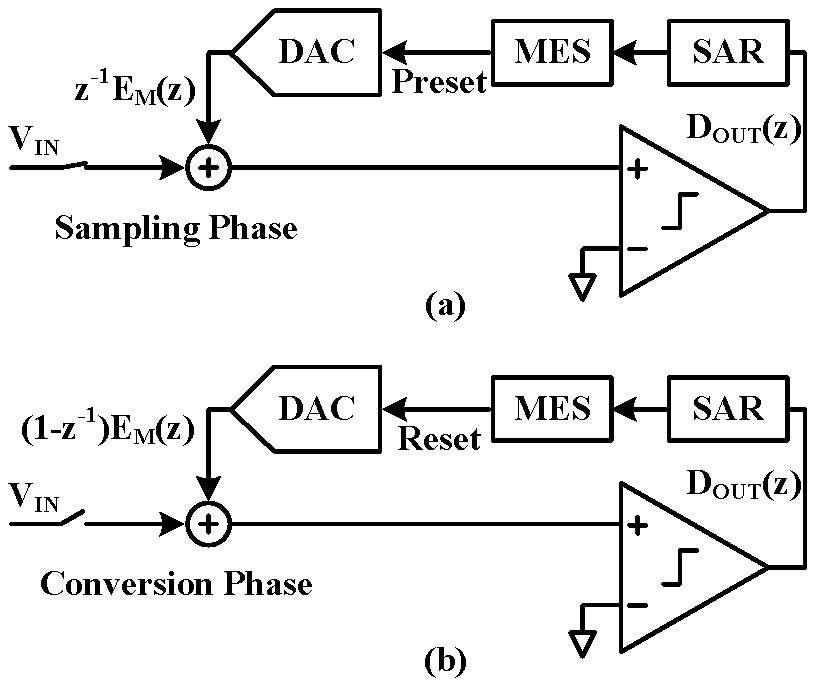To alleviate this loss, references [6,23,40] apply the DEM or DWA for the MSBs and MES for LSBs. These references can also mitigate the complexity of DEM-based implementations by applying DEM techniques to only some MSBs.

### 2. kT/C Noise

Sampling kT/C noise is one of the unshaped noises in NS-SAR ADCs. Even though oversampling relieves kT/C noise by OSR, the disadvantage is that increasing OSR limits the signal bandwidth. Fig. 19 shows sampling circuit and its equivalent noise modeling. The on-resistance of the sampling switch introduces the noise power spectral density (PSD) of $4kTR_{ON}$ and its equivalent noise bandwidth (ENBW) is $1/4R_{ON}C_{S}$. For a simple single-pole system, the total noise power ($\overline{v_{n}^{2}}$) can be expressed as the product of the PSD and ENBW .

##### (12)
$\overline{v_{n}^{2}}=4kTR_{ON}\times \frac{1}{4R_{ON}C_{S}}=\frac{kT}{C_{S}}$

Therefore, only the sampling capacitor appears in total noise power. Reference [46,47] propose a kT/C noise reduction scheme using feedback topology by decoupling the noise PSD and ENBW. Fig. 20(a) and (b) shows a sampling circuit with a single-stage and two-stage amplifier, respectively. They reduce total sampling noise as follows.

Note that $\gamma$ is the amplifier noise factor [46,47].

##### (13)
$\overline{v_{n1}^{2}}=\left(\frac{\gamma R_{L}}{R_{L}+R_{FB}}+\frac{1}{g_{m}R_{L}}+\frac{1}{g_{m}R_{FB}}\right)\times \frac{kT}{C_{S}}$
##### (14)
$\overline{v_{n2}^{2}}=\left(\gamma g_{m2}R_{L}+\frac{g_{m2}}{g_{m1}}+\frac{\gamma }{g_{m1}R_{L}}\right)\times \frac{kT}{C_{S}}$

If the amplifier gain, $g_{m,m1}$ is large, the noises can be approximate as

##### (15)
$\overline{v_{n1}^{2}}\approx \frac{\gamma R_{L}}{R_{L}+R_{FB}}\times \frac{kT}{C_{S}}$
##### (16)
$\overline{v_{n2}^{2}}\approx \gamma g_{m2}R_{L}\times \frac{kT}{C_{S}}$

It shows that the sampling noise can be adjusted by $R_{L},R_{FB},$ and $g_{m2}$ while $C_{S}$ remains constant.

References [22,30,46,48] introduce the active sampling kT/C noise canceling scheme as shown in Fig. 21. This scheme captures the noise on an additional series capacitor ($C_{N}$) which is placed between the preamplifier and the latch of the comparator. Then this noise would be canceled in the next phase. Although the additional series capacitor induces extra noise, this is attenuated by preamplifier gain. However, this noise cancellation cannot be employed directly in NS-SAR.

This is because the noise cancellation occurs inside the comparator, which causes the noise captured by $C_{N}$ to be shaped by NTF. To overcome this limitation, references [22,30] perform the noise cancellation in the pre-comparator feedback path as shown in Fig. 22. Although EF structure has been adopted to validate the pre-comparator kT/C noise cancellation, they can also be applied to CIFF structures. These techniques alleviate the burden of the ADC input driver by reducing the sampling capacitor.

##### Fig. 19. (a) Simple sampling circuit; (b) its equivalent noise modeling.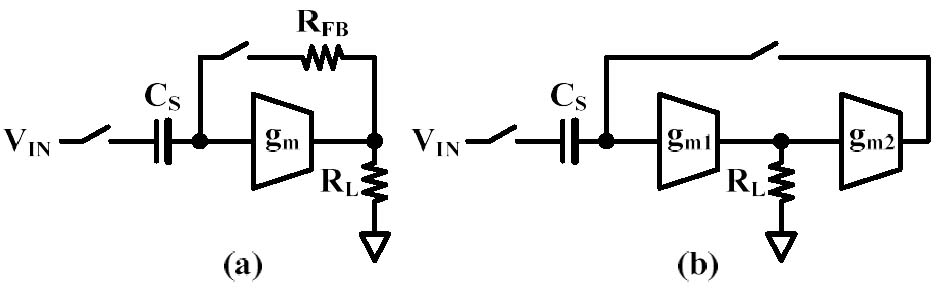##### Fig. 20. kT/C noise reduction using feedback topology with (a) single-stage; (b) two-stage amplifier[46,47].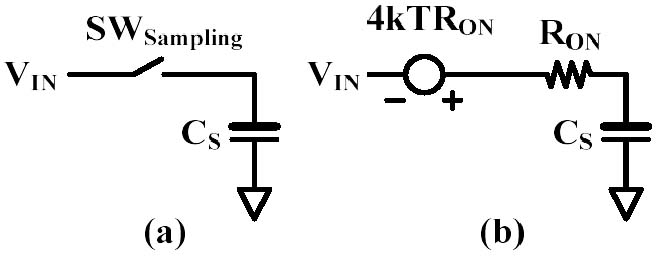##### Fig. 21. kT/C noise cancellation using series capacitor[46,48].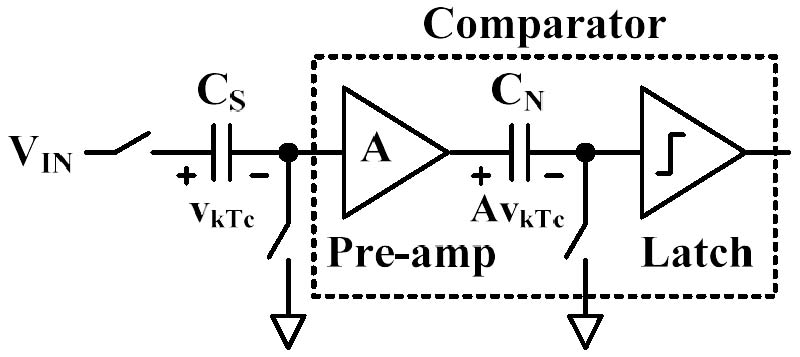##### Fig. 22. Pre-comparator kT/C noise cancellation[22,30].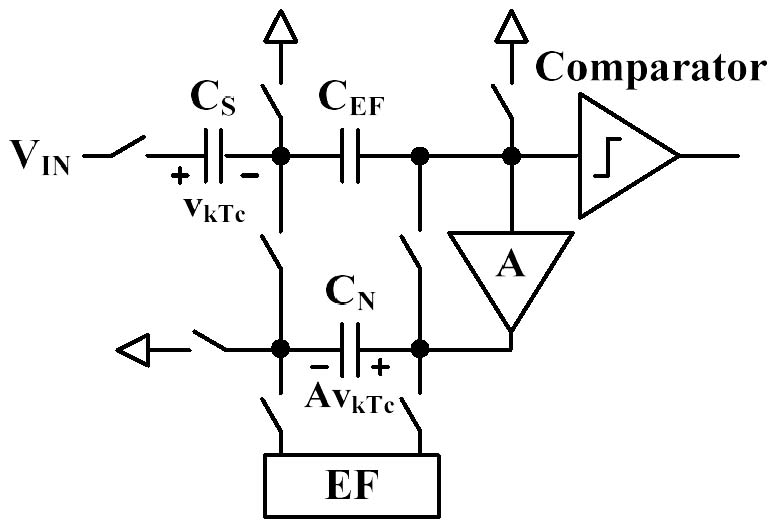## V. ADVANCED ARCHITECTURES (FUTURE TRENDS)

Even though the NS-SAR ADCs are a high-efficient and low-cost architecture, they still have inherent limitations, such as the challenge of driving large sampling capacitors for high-resolution NS-SAR and limited BW due to the oversampling. This section summarizes the advanced architectures employed in an effort to address these limitations.

### 1. Hybrid Architectures

In , the NS-SAR ADC is employed as a quantizer in continuous time (CT)-DSM ADC so as to achieve a power-efficient 3rd-order NS effect. It is implemented by means of a 1st-order CT-DSM ADC and a fully passive 2nd-order NS-SAR. Thanks to the 2nd-order NS-SAR, the overall loop filter can be simplified given that it needs only a single active amplifier to realize the 3rd-order NS effect.

In [50,51], the pipelined NS-SAR ADC is proposed to solve BW limitation. The 2nd-stage residue is summed with 1st-stage residue, which amplified by inter-stage residue amplifier. Then further quantized in 2nd-stage, realizing EF NS. Since the 2nd-stage integrates the residue during the 1st-stage sampling and conversion a high-speed pipeline operation is maintained.

In [32,39], the multi-stage noise-shaping (MASH) ADC is presented. Reference  implements NS-SAR assisted pipelined ADC, realizing 2-0 MASH. The 2nd-orer NS-SAR ADC shapes the inter-stage gain error and nonlinearities of pipelined ADC. It relaxes the gain sensitivity of conventional pipelined ADC. Reference  proposes 1-1 MASH structure using fully passive NS-SAR and VCO ADC alleviating the burden of driving large sampling capacitors. The proposed MASH ADC allows for the use of the low-resolution NS-SAR as a 1st-stage, allowing for small input capacitors while signal attenuation of passive NS-SAR is addressed by leveraging it to linearize the VCO.

### 2. Time-interleaved Architectures

The oversampling ADCs suffer from the limited BW due to the OSR. Time-interleaved (TI) architecture is a well-known technique for increasing BW. However, this TI strategy is not a straightforward solution for NS-SAR ADCs due to the residue process. Because the multiple channels (sub-ADCs) operate in parallel with overlapping the conversion cycles, the residue from the previous channel cannot be fed back to the adjacent channel directly as shown in Fig. 23(a). To apply the TI technique to NS-SAR ADC,  proposes the midway feedback which is multiple feedback to other channels as shown in Fig. 23(b). Thanks to the inherent delay in midway feedback, this allows high-order NTF to be realized. However, as the TI NS-SAR ADC in  is implemented on EF architecture, it requires a summing amplifier consuming large static power. Moreover, it shows mild NTF to ensure stability due to the gain of the amplifier being vulnerable to PVT variations. To overcome these drawbacks of the EF structure, [28,31] realizes the TI NS-SAR ADC using CIFF architecture.  uses the multi-input comparator as a feedforward summation and  adopts the passive summation using capacitor stacking scheme. Therefore, the CIFF-based TI NS-SAR ADCs show better power efficiency than EF-based structure due to unnecessariness of a static amplifier.

##### Fig. 23. (a) Inter-channel non-casual feedback; (b) midway feedback proposed in.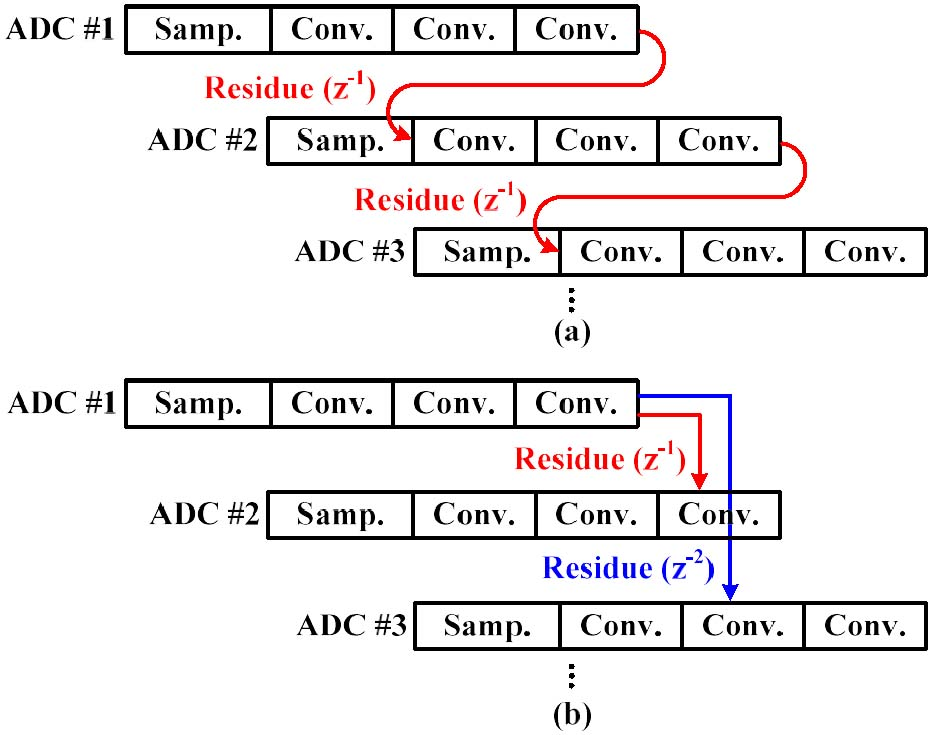## VI. CONCLUSION

In this paper, the fundamentals of NS-SAR ADCs are described. Additionally, the noise reduction techniques including the implementation of a loop filter, CDAC MES, and kT/C noise cancellation are also reviewed. The loop filters to realize NS effects are categorized as either active or passive topology with the advancements of each topology provided. Additional noise reduction techniques such as CDAC MES and kT/C cancellation to suppress the unshaped noise by NTF are presented. Advanced hybrid architectures capable of overcoming the inherent limitations of NS-SARs are also summarized.

Table 2 compares and summarizes the performance, feedback structure, loop filter implementation, and features of NS-SAR ADCs with chip measurement results.
##### Table 2. Comparison and summary
 Year Publication BW (MHz) Power ($\mu$W) OSR NS order SNDR (dB) SFDR (dB) FoMS (dB) FoMW (fJ/c.step) Structure Loop Filter Features 2012  JSSC 11 806 4 1 62.1 72.5 163.5 35 CIFF Active First CIFF NS-SAR ADC 2015  VLSIC 6.25 120.7 4 1 58 - 165.2 14.8 EF Passive First EF NS-SAR ADC 2016  ASSCC 8 252.9 4 2 64.9 - 169.9 11 EF Passive Passive gain with cap. stacking  ESSCIRC 0.125 61 8 1 74 95 167.1 59.6 CIFF Passive Multi-input comp. for relative gain  VLSIC 0.002 37.1 25 3 98 111.8 175.3 143 CIFF Active DEM, modulation dither effect  JSSC 0.001 15.7 500 1 101 105.1 179 85.6 CIFF Active DWA, MES 2017  CICC 1.75 70.5 8 1 68.1 84.8 172 9.7 CIFF Active Least squares estimation-based calibration  VLSIC 0.262 143 16 2 80 - 172.6 33.4 CIFF Passive Tri-level majority voting  VLSIC 25 2400 6 1 69.1 81.2 169.2 20.7 CIFF Passive Noise quantizer technique  ISSCC 5 460 13.2 1 79.7 92.6 180.1 5.8 CIFF Active Gain-enhanced dynamic amp.  CICC 0.25 257.8 20 3 83.4 96.5 173.3 42.6 CIFF Active Open-loop integrator, binary DEM 2018  ASSCC 0.05 60 16 2 72 78.7 161.2 184.4 CIFF Passive Majority voting, cycle DEM  ISSCC 0.625 84 8 2 79 89 177.7 9.2 EF Active NTF optimization  MWSCAS 0.002 74.2 32 1 78.8 87.6 153.1 2605.9 EF Active 2-C DAC 2019  ASSCC 5 108.7 4 2 68.2 84.6 174.8 5.2 CIFF Active Optimal 2-zeros & 2-poles, compiled layout  JSSC 50 13000 4 4 70.4 88 166.3 48 TI-EF Active Time-interleaved  CICC 2 2130 20 2 73.8 87.3 163.5 133 CIFF Passive Buffer-embedded  ISSCC 40 1250 4 1 66.6 77.4 171.6 9 CIFF Passive Passive signal-residue summation  ASSCC 0.625 130 8 2 71 81 167.8 35.9 EF Active Dynamic amp., ring amp. 2020  TCAS-I 0.625 70 8 2 74.6 - 174.1 12.8 EF Active Configurable band-pass, clock-controlled amp.  JSSC 0.1 120 10 4 87.6 102.8 176.8 30.6 Cas.-EF Active Multi-phase settling amp.  ISSCC 0.04 67.4 25 1 90.5 102.2 178.2 30.8 CIFF Passive Passive gain with cap. stacking, 2nd-order MES  MWSCAS 0.002 40.8 32 1 82.6 90.9 159.5 925.1 EF Active 2-C DAC, correlated double sampling  JSSC 0.625 107 8 2 83.8 94.3 181.5 6.8 CIFF Active Closed-loop dynamic amp.  TCAS-II 3.125 1240 16 2 77 90.1 171 34.3 CIFF Active Lossless integrator 2021  ASSCC 0.625 71 8 2 73.8 88.1 173.2 14.2 EF Active Open-loop V-T-V converter  ISSCC 80 2560 4 1 66.3 73.6 171.2 9.5 TI-CIFF Passive Coarse-fine segmentation  ISSCC 0.25 340 10 4 93.3 104.4 182 18 CIFF Active Cap. stacking, dynamic buffering  JSSC 0.625 119 8 3 84.8 103 182 6.7 EF-CIFF Active kT/C cancellation, floating inv. dynamic amp.  JSSC 50 8500 4 2 69.1 - 166.8 36.5 TI-CIFF Passive Fully dynamic  ISSCC 25 1260 8 2 75 92.1 178 5.5 CIFF Passive 2-0 MASH, NS-SAR assisted pipeline 2022  ISSCC 0.5 133.88 5 4 84.1 97 179.8 10.2 EF-CRFF Active EF-cascaded resonator FF, buffer-embedded  TCAS-II 0.625 113.02 16 2 79.3 90.4 176.7 12 EF Active Unity-gain buffer  JSSC 0.125 96 8 3 79.6 94.8 170.7 49.4 EF-CIFF Active Dither-based digital calibration  JSSC 1.1 160 11 1 71.5 81 169.9 23.7 CIFF Passive 1-1 MASH using NS-SAR & VCO  JSSC 5 8006 6 2 84.2 97.3 172.2 60.4 CIFF Active Buffer-embedded  JSSC 0.03125 7.3 16 2 80 98 176.3 14.3 CIFF Active Duty-cycled amp. digital-predicted MES

## ACKNOWLEDGMENTS

This work was supported in part by Chung-Ang University Research Grants in 2020, and in part by Institute of Information & Communications Technology Planning & Evaluation (IITP) grant funded by the Korea government (MSIT) (No. 2020-0-01294, Development of IoT based edge computing ultra-low power artificial intelligent processor).

## References

1
Fredenburg J. A., Flynn M. P., 2012, A 90-MS/s 11-MHz-Bandwidth 62-dB SNDR Noise-Shaping SAR ADC, IEEE Journal of Solid-State Circuits, Vol. 47, No. 12, pp. 2898-29042
Chen Z., Miyahara M., Matsuzawa A., 2015, A 9.35-ENOB, 14.8 fJ/conv.-step Fully-Passive Noise-Shaping SAR ADC, in 2015 Symposium on VLSI Circuits (VLSI Circuits), pp. C64-C653
Chen Z., Miyahara M., Matsuzawa A., 2016, A 2nd Order Fully-Passive Noise-Shaping SAR ADC with Embedded Passive Gain, in 2016 IEEE Asian Solid-State Circuits Conference (A-SSCC), pp. 309-3124
Guo W., Sun N., 2016, A 12b-ENOB 61µW Noise-Shaping SAR ADC with a Passive Integrator, in ESSCIRC Conference 2016: 42nd European Solid-State Circuits Conference, pp. 405-4085
Obata K., Matsukawa K., Miki T., Tsukamoto Y., Sushihara K., 2016, A 97.99 dB SNDR, 2 kHz BW, 37.1 µW Noise-Shaping SAR ADC with Dynamic Element Matching and Modulation Dither Effect, in 2016 IEEE Symposium on VLSI Circuits (VLSI-Circuits), pp. 1-26
Shu Y. S., Kuo L. T., Lo T. Y., 2016, An Oversampling SAR ADC With DAC Mismatch Error Shaping Achieving 105 dB SFDR and 101 dB SNDR Over 1 kHz BW in 55 nm CMOS, IEEE Journal of Solid-State Circuits, Vol. 51, No. 12, pp. 2928-29407
Garvik H., Wulff C., Ytterdal T., 2017, An 11.0 bit ENOB, 9.8 fJ/conv.-step Noise-Shaping SAR ADC Calibrated by Least Squares Estimation, in 2017 IEEE Custom Integrated Circuits Conference (CICC), pp. 1-48
Guo W., Zhuang H., Sun N., 2017, A 13b-ENOB 173dB-FoM 2nd-Order NS SAR ADC with Passive Integrators, in 2017 Symposium on VLSI Circuits, pp. C236-C2379
Lin Y. Z., Tsai C. H., Tsou S. C., Chu R. X., Lu C. H., 2017, A 2.4-mW 25-MHz BW 300-MS/s Passive Noise Shaping SAR ADC with Noise Quantizer Technique in 14-nm CMOS, in 2017 Symposium on VLSI Circuits, pp. C234-C23510
Liu C. C., Huang M. C., 2017, A 0.46mW 5MHz-BW 79.7dB-SNDR Noise-Shaping SAR ADC with Dynamic-Amplifier-based FIR-IIR Filter, in 2017 IEEE International Solid-State Circuits Conference (ISSCC), pp. 466-46711
Miyahara M., Matsuzawa A., 2017, An 84 dB Dynamic Range 62.5-625 kHz Bandwidth Clock-Scalable Noise-Shaping SAR ADC with Open-Loop Integrator using Dynamic Amplifier, in 2017 IEEE Custom Integrated Circuits Conference (CICC), pp. 1-412
Hwang Y., Song Y., Park J., Jeong D., 2018, A 0.6-to-1V 10k-to-100kHz BW 11.7b-ENOB Noise-Shaping SAR ADC for IoT Sensor Applications in 28-nm CMOS, in 2018 IEEE Asian Solid-State Circuits Conference (A-SSCC), pp. 247-24813
Li S., Qiao B., Gandara M., Sun N., 2018, A 13-ENOB 2nd-Order Noise-Shaping SAR ADC Realizing Optimized NTF zeros using an Error-Feedback Structure, in 2018 IEEE International Solid - State Circuits Conference - (ISSCC), pp. 234-23614
Shi L., Zhang Y., Wang Y., Kareppagoudr M., Sadollahi M., Temes G. C., 2018, A 13b-ENOB Noise Shaping SAR ADC with a Two-Capacitor DAC, in 2018 IEEE 61st International Midwest Symposium on Circuits and Systems (MWSCAS), pp. 153-15615
Garvik H., Wulff C., Ytterdal T., 2019, A 68 dB SNDR Compiled Noise-Shaping SAR ADC With On-Chip CDAC Calibration, in 2019 IEEE Asian Solid-State Circuits Conference (A-SSCC), pp. 193-19416
Jie L., Zheng B., Flynn M. P., 2019, A Calibration-Free Time-Interleaved Fourth-Order Noise-Shaping SAR ADC, IEEE Journal of Solid-State Circuits, Vol. 54, No. 12, pp. 3386-339517
Kim T., Chae Y., 2019, A 2MHz BW Buffer-Embedded Noise-Shaping SAR ADC Achieving 73.8dB SNDR and 87.3dB SFDR, in 2019 IEEE Custom Integrated Circuits Conference (CICC), pp. 1-418
Lin Y. Z., Lin C. Y., Tsou S. C., Tsai C. H., Lu C. H., 2019, 20.2 A 40MHz-BW 320MS/s Passive Noise-Shaping SAR ADC With Passive Signal-Residue Summation in 14nm FinFET, in 2019 IEEE International Solid- State Circuits Conference - (ISSCC), pp. 330-33219
Yoon J. S., Hong J., Kim J., 2019, A Digitally-Calibrated 70.98dB-SNDR 625kHz-Bandwidth Temperature-Tolerant 2nd-order Noise-Shaping SAR ADC in 65nm CMOS, in 2019 IEEE Asian Solid-State Circuits Conference (A-SSCC), pp. 195-19620
Jiao Z., et al , 2020, A Configurable Noise-Shaping Band-Pass SAR ADC With Two-Stage Clock-Controlled Amplifier, IEEE Transactions on Circuits and Systems I: Regular Papers, Vol. 67, No. 11, pp. 3728-373921
Jie L., Zheng B., Chen H. W., Flynn M. P., 2020, A Cascaded Noise-Shaping SAR Architecture for Robust Order Extension, IEEE Journal of Solid-State Circuits, Vol. 55, No. 12, pp. 3236-324722
Li S., 2020, A kT/C-Noise-Cancelled Noise-Shaping SAR ADC with a Duty-Cycled Amplifier, in 2020 IEEE 63rd International Midwest Symposium on Circuits and Systems (MWSCAS), pp. 758-76123
Liu J., Wang X., Gao Z., Zhan M., Tang X., Sun N., 2020, A 40kHz-BW 90dB-SNDR Noise-Shaping SAR with 4×Passive Gain and 2nd-Order Mismatch Error Shaping, in 2020 IEEE International Solid- State Circuits Conference - (ISSCC), pp. 158-16024
Shi L., Thaigarajan E., Singh R., Hancioglu E., Moon U. K., Temes G., 2020, Noise-Shaping SAR ADC Using a Two-Capacitor Digitally Calibrated DAC with 85.1 dB DR and 91 dB SFDR, in 2020 IEEE 63rd International Midwest Symposium on Circuits and Systems (MWSCAS), pp. 353-35625
Tang X., et al , 2020, A 13.5-ENOB, 107-μW Noise-Shaping SAR ADC With PVT-Robust Closed-Loop Dynamic Amplifier, IEEE Journal of Solid-State Circuits, Vol. 55, No. 12, pp. 3248-325926
Zhang Y., Liu S., Tian B., Zhu Y., Chan C. H., Zhu Z., 2020, A 2nd-Order Noise-Shaping SAR ADC With Lossless Dynamic Amplifier Assisted Integrator, IEEE Transactions on Circuits and Systems II: Express Briefs, Vol. 67, No. 10, pp. 1819-182327
Chen C. C., Hsieh C. C., 2021, A 12-ENOB Second-Order Noise Shaping SAR ADC with PVT-insensitive Voltage-Time-Voltage Converter, in 2021 IEEE Asian Solid-State Circuits Conference (A-SSCC), pp. 1-328
Lin C. Y., Lin Y. Z., Tsai C. H., Lu C. H., pp. 378-380, An 80MHz-BW 640MS/s Time-Interleaved Passive Noise-Shaping SAR ADC in 22nm FDSOI Process, in 2021 IEEE International Solid- State Circuits Conference (ISSCC), Vol. 202129
Liu J., Li D., Zhong Y., Tang X., Sun N., 2021, A 250kHz-BW 93dB-SNDR 4th-Order Noise-Shaping SAR Using Capacitor Stacking and Dynamic Buffering, in 2021 IEEE International Solid- State Circuits Conference (ISSCC), Vol. 64, pp. 369-37130
Wang T. H., Wu R., Gupta V., Tang X., Li S., 2021, A 13.8-ENOB Fully Dynamic Third-Order Noise-Shaping SAR ADC in a Single-Amplifier EF-CIFF Structure With Hardware-Reusing kT/C Noise Cancellation, IEEE Journal of Solid-State Circuits, Vol. 56, No. 12, pp. 3668-368031
Zhuang H., Liu J., Tang H., Peng X., Sun N., 2021, A Fully Dynamic Low-Power Wideband Time-Interleaved Noise-Shaping SAR ADC, IEEE Journal of Solid-State Circuits, Vol. 56, No. 9, pp. 2680-269032
Zhang H., Zhu Y., Chan C. H., Martins R. P., 2021, A 25MHz-BW 75dB-SNDR Inherent Gain Error Tolerance Noise-Shaping SAR-Assisted Pipeline ADC with Background Offset Calibration, in 2021 IEEE International Solid- State Circuits Conference (ISSCC), Vol. 64, pp. 380-38233
Wang T., Xie T., Liu Z., Li S., 2022, An 84dB-SNDR Low-OSR 4th-Order Noise-Shaping SAR with an FIA-Assisted EF-CRFF Structure and Noise-Mitigated Push-Pull Buffer-in-Loop Technique, in 2022 IEEE International Solid- State Circuits Conference (ISSCC), Vol. 65, pp. 418-42034
Yi P., Liang Y., Liu S., Xu N., Fang L., Hao Y., 2022, A 625kHz-BW, 79.3dB-SNDR Second-Order Noise-Shaping SAR ADC Using High-Efficiency Error-Feedback Structure, IEEE Transactions on Circuits and Systems II: Express Briefs, Vol. 69, No. 3, pp. 859-86335
Zhang et al Q., 2022, A 13-Bit ENOB Third-Order Noise-Shaping SAR ADC Employing Hybrid Error Control Structure and LMS-Based Foreground Digital Calibration, IEEE Journal of Solid-State Circuits, pp. 1-136
Jie L., et al , 2021, An Overview of Noise-Shaping SAR ADC: From Fundamentals to the Frontier, IEEE Open Journal of the Solid-State Circuits Society, Vol. 1, pp. 149-16137
Salgado G. M., O’Hare D., O’Connell I., 2021, Recent Advances and Trends in Noise Shaping SAR ADCs, IEEE Transactions on Circuits and Systems II: Express Briefs, Vol. 68, No. 2, pp. 545-54938
Shen L., Gao Z., Yang X., Shi W., Sun N., 2021, A 79dB-SNDR 167dB-FoM Bandpass ΔΣ ADC Combining N-Path Filter with Noise-Shaping SAR, in 2021 IEEE International Solid- State Circuits Conference (ISSCC), Vol. 64, pp. 382-38439
Chandrasekaran S. T., Bhanushali S. P., Pietri S., Sanyal A., 2022, OTA-Free 1-1 MASH ADC Using Fully Passive Noise-Shaping SAR & VCO ADC, IEEE Journal of Solid-State Circuits, Vol. 57, No. 4, pp. 1100-111140
Guo Y., Jin J., Liu X., Zhou J., 2022, A 60-MS/s 5-MHz BW Noise-Shaping SAR ADC With Integrated Input Buffer Achieving 84.2-dB SNDR and 97.3-dB SFDR Using Dynamic Level-Shifting and ISI-Error Correction, IEEE Journal of Solid-State Circuits, pp. 1-1241
Li H., Shen Y., Xin H., Cantatore E., Harpe P., 2022, A 7.3-uW 13-ENOB 98-dB SFDR Noise-Shaping SAR ADC With Duty-Cycled Amplifier and Mismatch Error Shaping, IEEE Journal of Solid-State Circuits, Vol. 57, No. 7, pp. 2078-208942
Murmann B., 2022, ADC Performance Survey 1997-2022, https://web.stanford.edu/~murmann/adcsurvey.html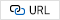43
Ahmed I., Mulder J., Johns D. A., 2010, A Low-Power Capacitive Charge Pump Based Pipelined ADC, IEEE Journal of Solid-State Circuits, Vol. 45, No. 5, pp. 1016-102744
Plassche R. J. V. D., 1976, Dynamic element matching for high-accuracy monolithic D/A converters, IEEE Journal of Solid-State Circuits, Vol. 11, No. 6, pp. 795-80045
Baird R. T., Fiez T. S., 1995, Linearity Enhancement of Multibit Delta Sigma A/D and D/A Converters using Data Weighted Averaging, IEEE Transactions on Circuits and Systems II: Analog and Digital Signal Processing, Vol. 42, No. 12, pp. 753-76246
Kapusta R., Zhu H., Lyden C., 2014, Sampling Circuits That Break the kT/C Thermal Noise Limit, IEEE Journal of Solid-State Circuits, Vol. 49, No. 8, pp. 1694-170147
Li Z., et al , 2020, A SAR ADC with Reduced kT/C Noise by Decoupling Noise PSD and BW, in 2020 IEEE Symposium on VLSI Circuits, pp. 1-248
Liu J., Tang X., Zhao W., Shen L., Sun N., 2020, A 13-bit 0.005-mm2 40-MS/s SAR ADC With kT/C Noise Cancellation, IEEE Journal of Solid-State Circuits, Vol. 55, No. 12, pp. 3260-327049
Liu J., Li S., Guo W., Wen G., Sun N., 2018, A 0.029mm2 17-FJ/Conv.-Step CT Delta-Sigma ADC with 2nd-Order Noise-Shaping SAR Quantizer, in 2018 IEEE Symposium on VLSI Circuits, pp. 201-20250
Song Y., Zhu Y., Chan C., Geng L., Martins R. P., 2018, A 77dB SNDR 12.5MHz Bandwidth 0–1 MASH ∑Δ ADC Based on the Pipelined-SAR Structure, in 2018 IEEE Symposium on VLSI Circuits, pp. 203-20451
Song Y., Zhu Y., Chan C. H., Martins R. P., 2020, A 2.56mW 40MHz-Bandwidth 75dB-SNDR Partial-Interleaving SAR-Assisted NS Pipeline ADC With Background Inter-Stage Offset Calibration, in 2020 IEEE International Solid- State Circuits Conference - (ISSCC), pp. 164-166Kiho Seong received the B.S. and M.S. degrees at School of Electrical and Electronics Engineering from Chung-Ang University (CAU), Seoul, Korea, in 2018 and 2020, respectively. He is currently working toward the Ph.D. degree in electrical and electronics engineering. His research interests include high-speed and high-resolution analog-to-digital converter (ADC).

Jae-Soub Han received the B.S. and M.S. degrees at School of Electrical and Electronics Engineering from Chung-Ang University (CAU), Seoul, Korea, in 2019 and 2021, respectively. He is currently working toward the Ph.D. degree in electrical and electronics engineering. His research interests include high speed direct digital frequency synthesizer (DDFS) and low-power phase-locked loop (PLL).

Sung-Eun Kim was born in South Korea in October 1978. He received the B.S. degree in electrical and computer engineering from Hanyang University, Seoul, South Korea, in 2002, and the M.S. degree in electrical engineering from the Korea Advanced Institute of Science and Technology (KAIST), Daejeon, South Korea, in 2004. Since March 2004, he has been with the Electronics and Telecommunications Research Institute (ETRI), Daejeon, where he is currently a Principal Researcher. He has been primarily involved in researching analog circuit design for human body communications and power management of energy harvesting systems.

Yong Shim received the B.S. and M.S. degrees in electronics engi-neering from Korea University, in 2004 and 2006, respectively, and the Ph.D. degree from the School of Electrical and Computer Engineering, Purdue University, West Lafayette, IN, in 2018. He was a Memory Interface Designer with Samsung Electronics, Hwaseong, from 2006 to 2013. At Samsung, he has worked on the design and development of a memory interface for synchronous DRAMs (DDR1 and DDR4). He is currently an Assistant Professor with Chung-Ang University. Prior to joining Chung-Ang University, in 2020, he was an SRAM Designer with Intel Corporation, Hillsboro, OR, from 2018 to 2020, where he was involved in designing circuits for super-scaled next-generation SRAM cache design. His research interests include neuromorphic hardware and algorithm, in-memory computing, robust memory interface design, as well as emerging devices (RRAM, MRAM, and STO) based unconventional computing models.

Kwang-Hyun Baek received the B.S. and M.S. degrees from Korea University, Seoul, Korea, in 1990 and 1998, respectively. He received the Ph.D. degree in electrical engineering from the University of Illinois at Urbana-Champaign (UIUC), IL, USA, in 2002. From 2000 to 2006, he was with the Department of High-Speed Mixed-Signal ICs as a senior scientist at Rockwell Scientific Company, formerly Rockwell Science Center (RSC), Thousand Oaks, CA, USA. At RSC, he was involved in development of high-speed data converters (ADC/DAC) and direct digital frequency synthesizers (DDFS). He was also with Samsung Electronics from 1990 to 1996. Since 2006 he has been with the School of Electrical and Electronics Engineering, Chung-Ang University (CAU), Seoul, Korea, where he is a faculty member. His research interests include high-performance analog and digital circuits such as low-power ADCs, high-speed DACs, hybrid frequency synthesizers (PLLs, DDFSs), high-speed interface circuits (CDRs, SerDes), PMIC, and near threshold-voltage (NTV) circuits.